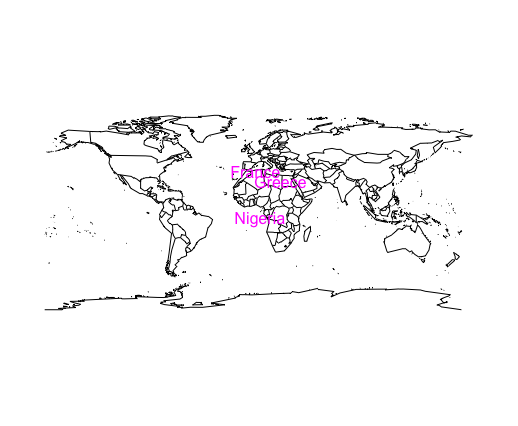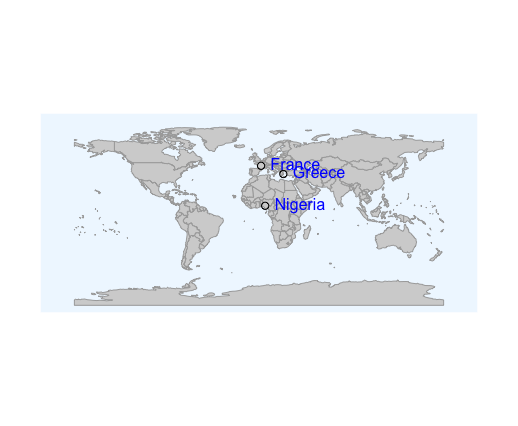Open in App
Not now

# Adding labels to points plotted on world map in R

• Last Updated : 17 Jun, 2021

In this article, we are going to see how to add labels to points plotted on the world map in R Programming Language.

### Method 1: Using maps package

Maps: The “maps” package in R is used to draw and display geographical maps. It contains various databases for denoting countries, continents and seas. The package can be installed and loaded into the working space using the following command :

`install.packages("maps")`

The package contains the ‘world’ database, which contains descriptive images of continents and it no longer contains lakes and lake islands. The map function of this package is used to draw lines and polygons as specified by a map database, which incorporates the geographical map.

map(database = “world”)

The data can be specified in the form of latitudes and longitudes and the names of the cities. The text can then be annotated over this plot using the text() method. It can be customized with various attributes to improve readability and enhance the graphics.

## R

 `# Load required libraries``library``(maps)`` ` `# capturing data of cities``data_frame <- ``data.frame``(name = ``c``(``"Greece"` `, ``"France"` `, ``"Nigeria"``),``                         ``latitude = ``c``(38.0,46.0,7.0),``                         ``longitude = ``c``(23.7,2.0,6.0))``map``(database = ``"world"``)`` ` `# marking points on map``text``(x = data_frame\$longitude, y = data_frame\$latitude, ``     ``data_frame\$name, pos = 1, col = ``"magenta"``)`

Output### Method 2: Using rworldmap Package

The “rworldmap” can be used for mapping global data and also enables the mapping of country-level and gridded user datasets. It can be downloaded and installed into the working space by the following command :

`install.packages("rworldmap")`

The getMap() method can be used to access maps stored in the package.

getMap(resolution = “coarse”)

The plot() method is used to plot the world map over an opened graphical device. It can be customized to add color to the plot and specify the dimensions of the plotting device.

plot (worldMap , col = , border = )

The points() can be added by the specification of longitude, latitude coordinates. The text() method can be used to annotate these points using the text() method.

Syntax: text ( x , y , names, col = )

Arguments :

• x, y: The x and y coordinates respectively.
• names: The names to be assigned equivalent to the x and y coordinates.
• col: The colour used to annotate the points.

## R

 `# load library``library``(rworldmap)`` ` `# get world map``worldmap <- ``getMap``(resolution = ``"coarse"``)`` ` `# plot world map``plot``(worldmap, col = ``"lightgrey"``, ``     ``fill = T, border = ``"darkgray"``,``     ``xlim = ``c``(-180, 180), ylim = ``c``(-90, 90),``     ``bg = ``"aliceblue"``     ``)`` ` `# defining data frame``data_frame <- ``data.frame``(name = ``c``(``"Greece"` `, ``"France"` `, ``"Nigeria"``),``                         ``latitude = ``c``(38.0,46.0,7.0),``                         ``longitude = ``c``(23.7,2.0,6.0))`` ` `# marking the points in the map ``points``(x = data_frame\$longitude, y = data_frame\$latitude)`` ` `# adding text to map ``text``(x = data_frame\$longitude, y = data_frame\$latitude, ``     ``data_frame\$name, pos = 4, col = ``"blue"``)`

OutputMy Personal Notes arrow_drop_up